# 生成式深度学习• 用变分自编码器生成图像
• GAN 简介
• 如何用 GAN 生成图像
• 比较 VAE 与 GAN 的异同
• CGAN、DCGAN 简介

## 8.1 用变分自编码器生成图像

### 8.1.1 自编码器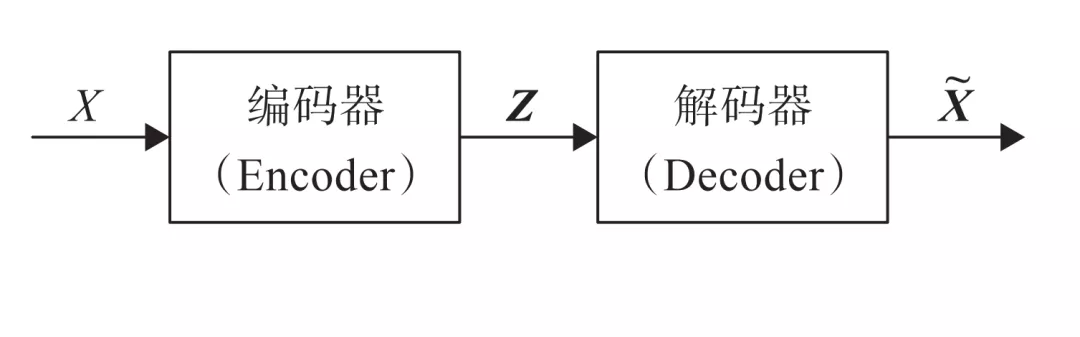### 8.1.2 变分自编码器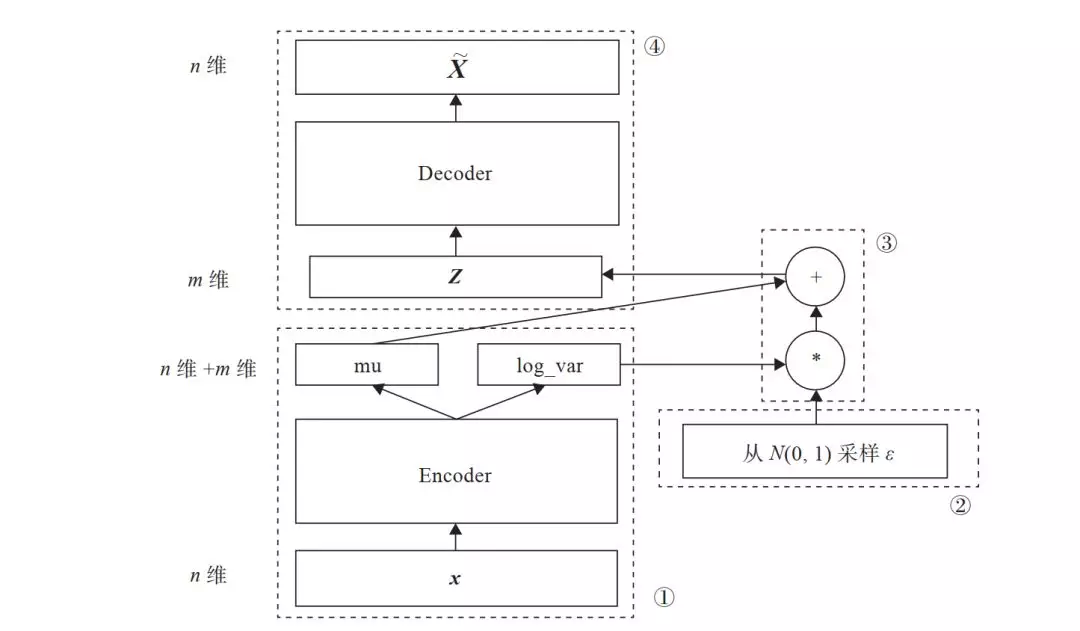**Z = mu + exp(log_var)\*（8-1）**

Z 是从潜在空间抽取的一个向量，Z 通过解码器生成一个样本 X~，这是模块④的功能。

1. 生成的新图像与原图像的相似度；
2. 隐含空间的分布与正态分布的相似度。

https://arxiv.org/pdf/1606.05908.pdf

# 定义重构损失函数及 KL 散度reconst_loss = F.binary_cross_entropy(x_reconst, x, size_average=False)kl_div = - 0.5 * torch.sum(1 + log_var - mu.pow(2) - log_var.exp())#两者相加得总损失loss= reconst_loss+ kl_div

### 8.1.3 用变分自编码器生成图像

1. 导入必要的包。

import osimport torchimport torch.nn as nnimport torch.nn.functional as Fimport torchvisionfrom torchvision import transformsfrom torchvision.utils import save_image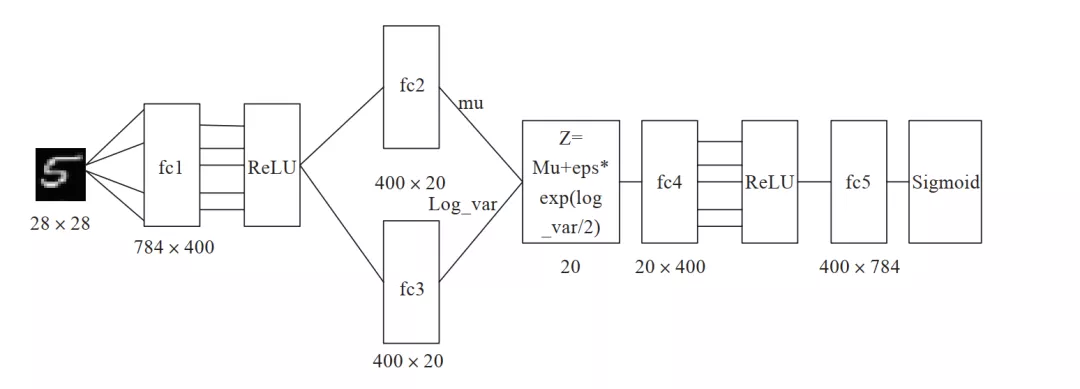1. 定义一些超参数。

image_size = 784h_dim = 400z_dim = 20num_epochs = 30batch_size = 128learning_rate = 0.001
1. 对数据集进行预处理，如转换为 Tensor，把数据集转换为循环、可批量加载的数据集。

# 下载 MNIST 训练集，这里因已下载，故 download=False# 如果需要下载，设置 download=True 将自动下载dataset = torchvision.datasets.MNIST(root='data',                                     train=True,                                     transform=transforms.ToTensor(),                                     download=False)                                          shuffle=True) #数据加载data_loader = torch.utils.data.DataLoader(dataset=dataset,                                          batch_size=batch_size,
1. 构建 AVE 模型，主要由 Encode 和 Decode 两部分组成。

# 定义 AVE 模型class VAE(nn.Module):    def __init__(self, image_size=784, h_dim=400, z_dim=20):        super(VAE, self).__init__()        self.fc1 = nn.Linear(image_size, h_dim)        self.fc2 = nn.Linear(h_dim, z_dim)        self.fc3 = nn.Linear(h_dim, z_dim)        self.fc4 = nn.Linear(z_dim, h_dim)        self.fc5 = nn.Linear(h_dim, image_size)     def encode(self, x):        h = F.relu(self.fc1(x))        return self.fc2(h), self.fc3(h) #用 mu，log_var 生成一个潜在空间点 z，mu，log_var 为两个统计参数，我们假设#这个假设分布能生成图像。def reparameterize(self, mu, log_var):        std = torch.exp(log_var/2)        eps = torch.randn_like(std)        return mu + eps * std     def decode(self, z):        h = F.relu(self.fc4(z))        return F.sigmoid(self.fc5(h))     def forward(self, x):        mu, log_var = self.encode(x)        z = self.reparameterize(mu, log_var)        x_reconst = self.decode(z)        return x_reconst, mu, log_var
1. 选择 GPU 及优化器。

# 设置 PyTorch 在哪块 GPU 上运行，这里假设使用序号为 1 的这块 GPU.torch.cuda.set_device(1)device = torch.device('cuda' if torch.cuda.is_available() else 'cpu')model = VAE().to(device)optimizer = torch.optim.Adam(model.parameters(), lr=learning_rate)
1. 训练模型，同时保存原图像与随机生成的图像。

with torch.no_grad():        # 保存采样图像，即潜在向量 Z 通过解码器生成的新图像        z = torch.randn(batch_size, z_dim).to(device)        out = model.decode(z).view(-1, 1, 28, 28)        save_image(out, os.path.join(sample_dir, 'sampled-{}.png'.format(epoch+1)))         # 保存重构图像，即原图像通过解码器生成的图像        out, _, _ = model(x)        x_concat = torch.cat([x.view(-1, 1, 28, 28), out.view(-1, 1, 28, 28)], dim=3)        save_image(x_concat, os.path.join(sample_dir, 'reconst-{}.png'.format(epoch+1)))'
1. 展示原图像及重构图像。

reconsPath = './ave_samples/reconst-30.png'Image = mpimg.imread(reconsPath)plt.imshow(Image) # 显示图像plt.axis('off') # 不显示坐标轴plt.show()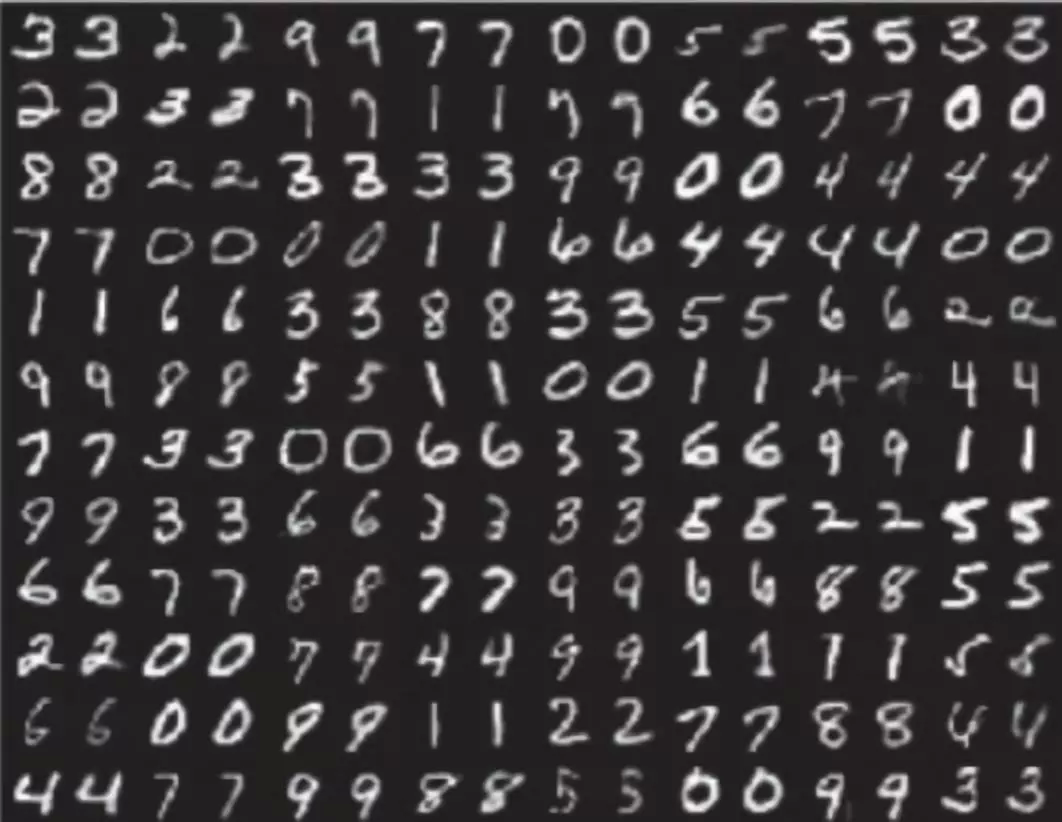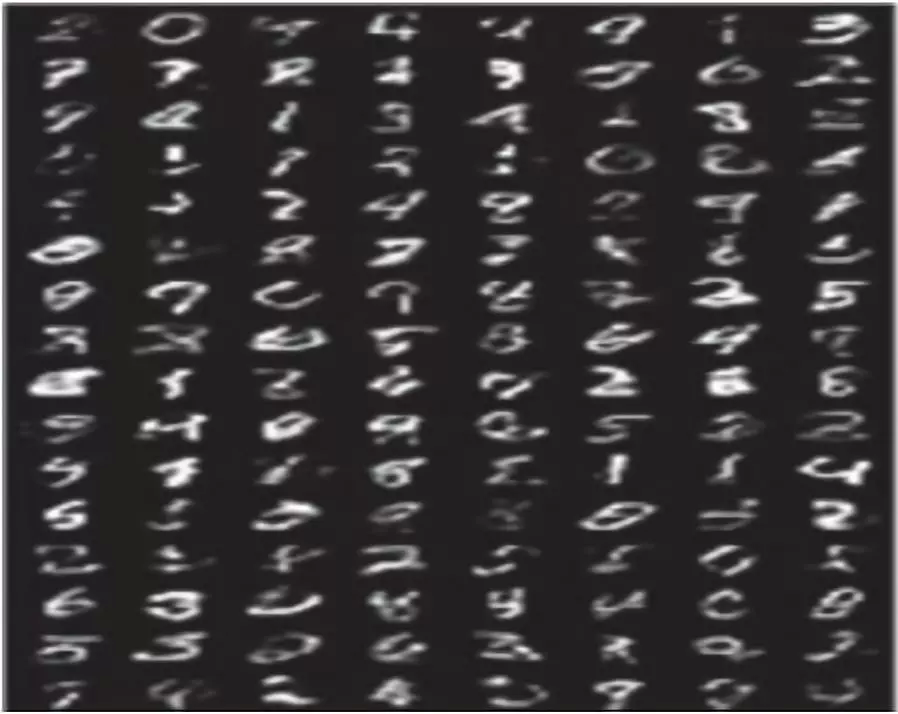1. 显示由潜在空间点 Z 生成的新图像。

genPath = './ave_samples/sampled-30.png'Image = mpimg.imread(genPath)plt.imshow(Image) # 显示图像plt.axis('off') # 不显示坐标轴plt.show()

## 8.2 GAN 简介

8.1 节介绍了基于自动编码器的 AVE，根据这个网络可以生成新的图像。本节我们将介绍另一种生成式网络，它是基于博弈论的，所以又称为生成式对抗网络 ( Generative Adversarial Nets，GAN )。它是 2014 年由 Ian Goodfellow 提出的，它要解决的问题是如何从训练样本中学习出新样本，训练样本就是图像就生成新图像，训练样本是文章就输出新文章等。

GAN 既不依赖标签来优化，也不是根据对结果奖惩来调整参数。它是依据生成器和判别器之间的博弈来不断优化。打个不一定很恰当的比喻，就像一台验钞机和一台制造假币的机器之间的博弈，两者不断博弈，博弈的结果假币越来越像真币，直到验钞机无法识别一张货币是假币还是真币为止。这样说，还是有点抽象，接下来我们将从多个侧面进行说明。

### 8.2.1 GAN 架构

VAE 利用潜在空间，可以生成连续的新图像，不过因损失函数采用像素间的距离，所以图像有点模糊。那能否生成更清晰的新图像呢？可以的，这里采用 GAN 替换 VAE 的潜在空间，它能够迫使生成图像与真实图像在统计上几乎无法区别的逼真合成图像。

GAN 的直观理解，可以想象一个名画伪造者想伪造一幅达芬奇的画作，开始时，伪造者技术不精，但他将自己的一些赝品和达芬奇的作品混在一起，请一个艺术商人对每一幅画进行真实性评估，并向伪造者反馈，告诉他哪些看起来像真迹、哪些看起来不像真迹。

1. 生成器网络：它一个潜在空间的随机向量作为输入，并将其解码为一张合成图像。
2. 判别器网络：以一张图像 ( 真实的或合成的均可 ) 作为输入，并预测该图像来自训练集还是来自生成器网络。图 8-6 为其架构图。

GAN 的优化过程不像通常的求损失函数的最小值，而是保持生成与判别两股力量的动态平衡。因此，其训练过程要比一般神经网络难很多。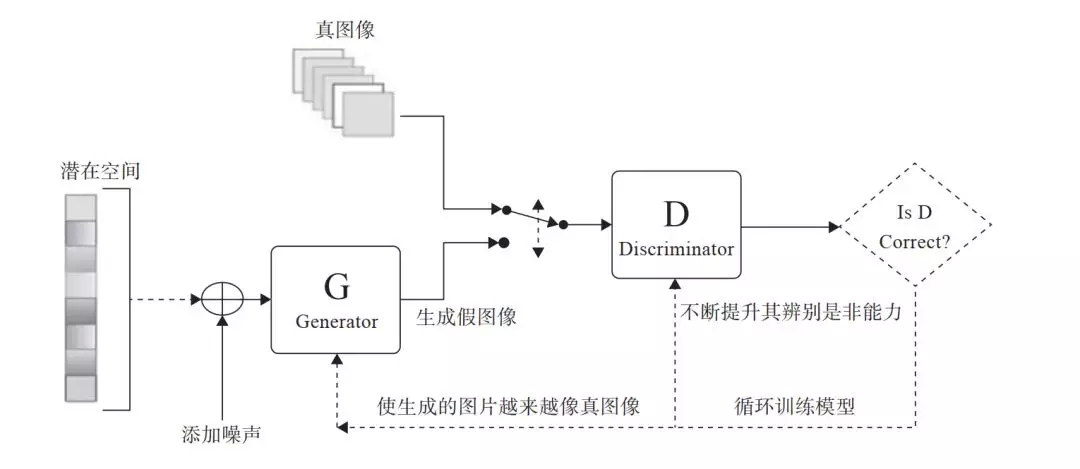### 8.2.2 GAN 的损失函数

# 定义判断器对真图像的损失函数outputs = D(images)d_loss_real = criterion(outputs, real_labels)real_score = outputs # 定义判别器对假图像（即由潜在空间点生成的图像）的损失函数z = torch.randn(batch_size, latent_size).to(device)fake_images = G(z)outputs = D(fake_images)d_loss_fake = criterion(outputs, fake_labels)fake_score = outputs            # 得到判别器总的损失函数d_loss = d_loss_real + d_loss_fake

z = torch.randn(batch_size, latent_size).to(device)fake_images = G(z)outputs = D(fake_images)         g_loss = criterion(outputs, real_labels)

## 8.3 用 GAN 生成图像

### 8.3.1 判别器

# 构建判断器D = nn.Sequential(    nn.Linear(image_size, hidden_size),    nn.LeakyReLU(0.2),    nn.Linear(hidden_size, hidden_size),    nn.LeakyReLU(0.2),    nn.Linear(hidden_size, 1),    nn.Sigmoid())

### 8.3.2 生成器

# 构建生成器，这个相当于 AVE 中的解码器G = nn.Sequential(    nn.Linear(latent_size, hidden_size),    nn.ReLU(),    nn.Linear(hidden_size, hidden_size),    nn.ReLU(),    nn.Linear(hidden_size, image_size),    nn.Tanh())

### 8.3.3 训练模型

for epoch in range(num_epochs):    for i, (images, _) in enumerate(data_loader):        images = images.reshape(batch_size, -1).to(device)         # 定义图像是真或假的标签        real_labels = torch.ones(batch_size, 1).to(device)        fake_labels = torch.zeros(batch_size, 1).to(device)         #==================================================================== #        #                           训练判别器                                  #        #==================================================================== #         # 定义判别器对真图像的损失函数        outputs = D(images)        d_loss_real = criterion(outputs, real_labels)        real_score = outputs         # 定义判别器对假图像（即由潜在空间点生成的图像）的损失函数        z = torch.randn(batch_size, latent_size).to(device)        fake_images = G(z)        outputs = D(fake_images)        d_loss_fake = criterion(outputs, fake_labels)        fake_score = outputs                 # 得到判别器总的损失函数        d_loss = d_loss_real + d_loss_fake         # 对生成器、判别器的梯度清零                reset_grad()        d_loss.backward()        d_optimizer.step()         #==================================================================== #        #                           训练生成器                                  #        #==================================================================== #         # 定义生成器对假图像的损失函数，这里我们要求        #判别器生成的图像越来越像真图片，故损失函数中        #的标签改为真图像的标签，即希望生成的假图像，        #越来越靠近真图像        z = torch.randn(batch_size, latent_size).to(device)        fake_images = G(z)        outputs = D(fake_images)          g_loss = criterion(outputs, real_labels)         # 对生成器、判别器的梯度清零        #进行反向传播及运行生成器的优化器        reset_grad()        g_loss.backward()        g_optimizer.step()         if (i+1) % 200 == 0:            print('Epoch [{}/{}], Step [{}/{}], d_loss: {:.4f}, g_loss: {:.4f}, D(x): {:.2f}, D(G(z)): {:.2f}'                  .format(epoch, num_epochs, i+1, total_step, d_loss.item(), g_loss.item(),                          real_score.mean().item(), fake_score.mean().item()))     # 保存真图像    if (epoch+1) == 1:        images = images.reshape(images.size(0), 1, 28, 28)        save_image(denorm(images), os.path.join(sample_dir, 'real_images.png'))     # 保存假图像    fake_images = fake_images.reshape(fake_images.size(0), 1, 28, 28)    save_image(denorm(fake_images), os.path.join(sample_dir, 'fake_images-{}.png'.format(epoch+1))) # 保存模型torch.save(G.state_dict(), 'G.ckpt')torch.save(D.state_dict(), 'D.ckpt')

### 8.3.4 可视化结果

reconsPath = './gan_samples/fake_images-200.png'Image = mpimg.imread(reconsPath)plt.imshow(Image) # 显示图片plt.axis('off') # 不显示坐标轴plt.show()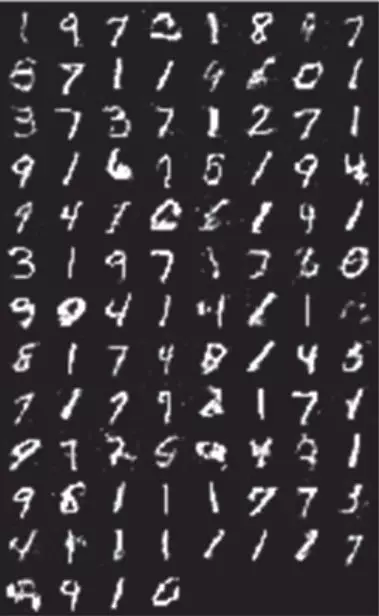## 8.4 VAE 与 GAN 的优缺点

VAE 和 GAN 都是生成模型 ( Generative Model )。所谓生成模型，即能生成样本的模型，利用这类模型，我们可以完成图像自动生成 ( 采样 )、图像信息补全等工作。

VAE 是利用已有图像在编码器生成潜在向量，这个向量在服从高斯分布的情况下很好地保留了原图像的特征，在解码器得到的图片会更加的合理与准确。

VAE 适合于学习具有良好结构的潜在空间，潜在空间有比较好的连续性，其中存在一些有特定意义的方向。VAE 能够捕捉到图像的结构变化 ( 倾斜角度、圈的位置、形状变化、表情变化等 )。这也是 VAE 的一大优点，它有显式的分布，能够容易地可视化图像的分布，具体如图 8-8 所示。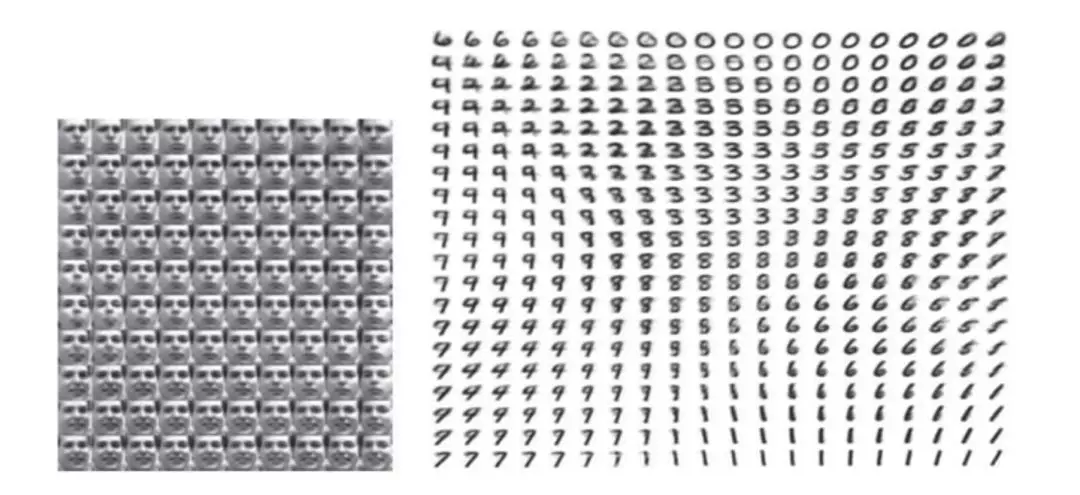GAN 生成的潜在空间可能没有良好结构，但 GAN 生成的图像一般比 VAE 的更清晰。

GAN 生成器的损失函数 ( Loss ) 依赖于判别器 Loss 后向传递，而不是直接来自距离，因而若判别器总是能准确地判别出真假，则向后传递的信息就非常少, 导致生成器无法形成自己的 Loss，这是 GAN 比较难训练的原因。当然，针对这一不足，近些年人们采用一个新的距离定义 ( Wasserstein Distance ) 应用于判别器，而不是原型中简单粗暴的对真伪样本的分辨正确的概率。

1. GAN 生成的效果优于 VAE。
2. GAN 比 VAE 更难训练。

## 8.5 ConditionGAN

AVE 和 GAN 都能基于潜在空间的随机向量 z 生成新图片，GAN 生成的图像比 AVE 的更清晰，质量更好些。不过它们生成的都是随机的，无法预先控制你要生成的哪类或哪个数。

### 8.5.1 CGAN 的架构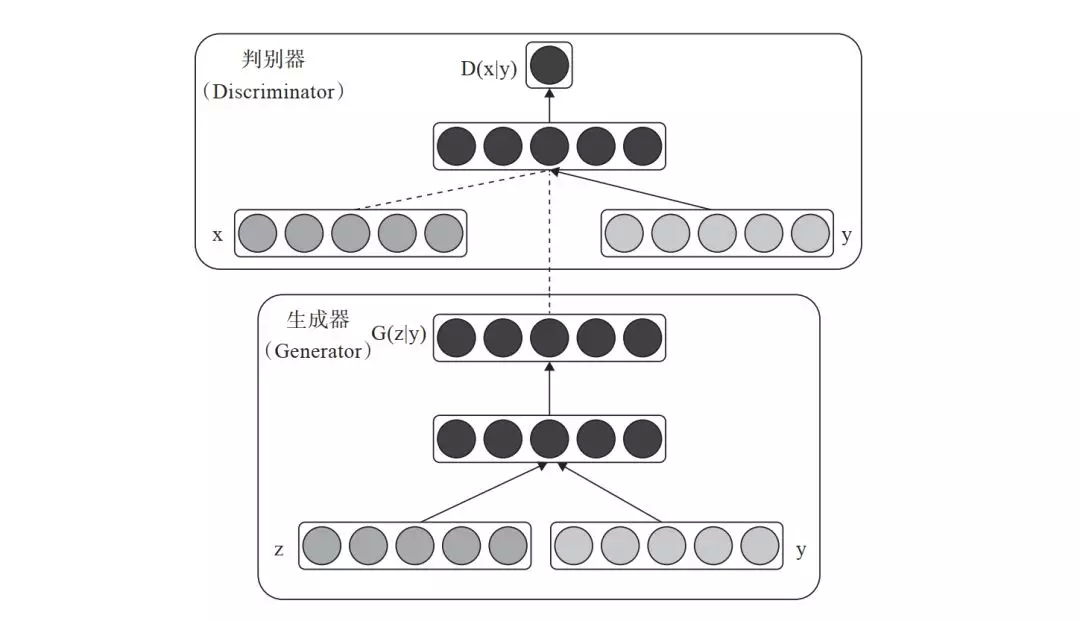### 8.5.2 CGAN 生成器

class Generator(nn.Module):    def __init__(self):        super().__init__()         self.label_emb = nn.Embedding(10, 10)         self.model = nn.Sequential(            nn.Linear(110, 256),            nn.LeakyReLU(0.2, inplace=True),            nn.Linear(256, 512),            nn.LeakyReLU(0.2, inplace=True),            nn.Linear(512, 1024),            nn.LeakyReLU(0.2, inplace=True),            nn.Linear(1024, 784),            nn.Tanh()        )     def forward(self, z, labels):        z = z.view(z.size(0), 100)        c = self.label_emb(labels)        x = torch.cat([z, c], 1)        out = self.model(x)        return out.view(x.size(0), 28, 28){1}

### 8.5.3 CGAN 判别器

class Discriminator(nn.Module):    def __init__(self):        super().__init__()         self.label_emb = nn.Embedding(10, 10)         self.model = nn.Sequential(            nn.Linear(794, 1024),            nn.LeakyReLU(0.2, inplace=True),            nn.Dropout(0.4),            nn.Linear(1024, 512),            nn.LeakyReLU(0.2, inplace=True),            nn.Dropout(0.4),            nn.Linear(512, 256),            nn.LeakyReLU(0.2, inplace=True),            nn.Dropout(0.4),            nn.Linear(256, 1),            nn.Sigmoid()        )     def forward(self, x, labels):        x = x.view(x.size(0), 784)        c = self.label_emb(labels)        x = torch.cat([x, c], 1)        out = self.model(x)        return out.squeeze(){1}

### 8.5.4 CGAN 损失函数

#定义判别器对真图像的损失函数real_validity = D(images, labels)d_loss_real = criterion(real_validity, real_labels)# 定义判别器对假图像（即由潜在空间点生成的图像）的损失函数z = torch.randn(batch_size, 100).to(device)fake_labels = torch.randint(0,10,(batch_size,)).to(device)fake_images = G(z, fake_labels)fake_validity = D(fake_images, fake_labels)d_loss_fake = criterion(fake_validity, torch.zeros(batch_size).to(device))#CGAN 总的损失值d_loss = d_loss_real + d_loss_fake

### 8.5.5 CGAN 可视化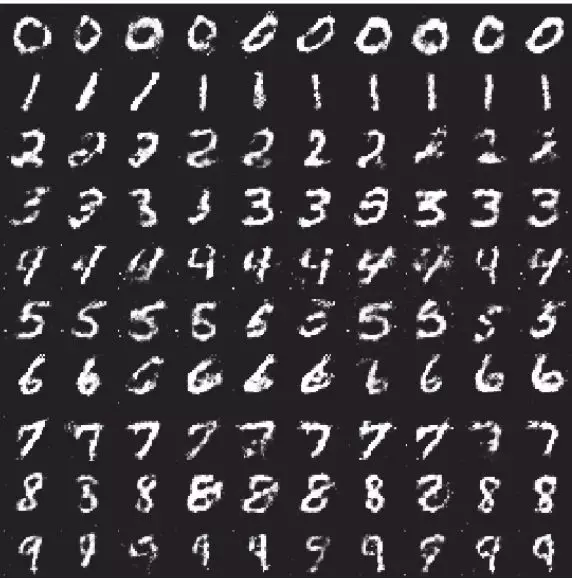from torchvision.utils import make_gridz = torch.randn(100, 100).to(device)labels = torch.LongTensor([i for i in range(10) for _ in range(10)]).to(device) images = G(z, labels).unsqueeze(1)grid = make_grid(images, nrow=10, normalize=True)fig, ax = plt.subplots(figsize=(10,10))ax.imshow(grid.permute(1, 2, 0).detach().cpu().numpy(), cmap='binary')ax.axis('off')

### 8.5.6 查看指定标签的数据

def generate_digit(generator, digit):    z = torch.randn(1, 100).to(device)    label = torch.LongTensor([digit]).to(device)    img = generator(z, label).detach().cpu()    img = 0.5 * img + 0.5    return transforms.ToPILImage()(img)generate_digit(G, 8)

### 8.5.7 可视化损失值

writer.add_scalars('scalars', {'g_loss': g_loss, 'd_loss': d_loss}, step)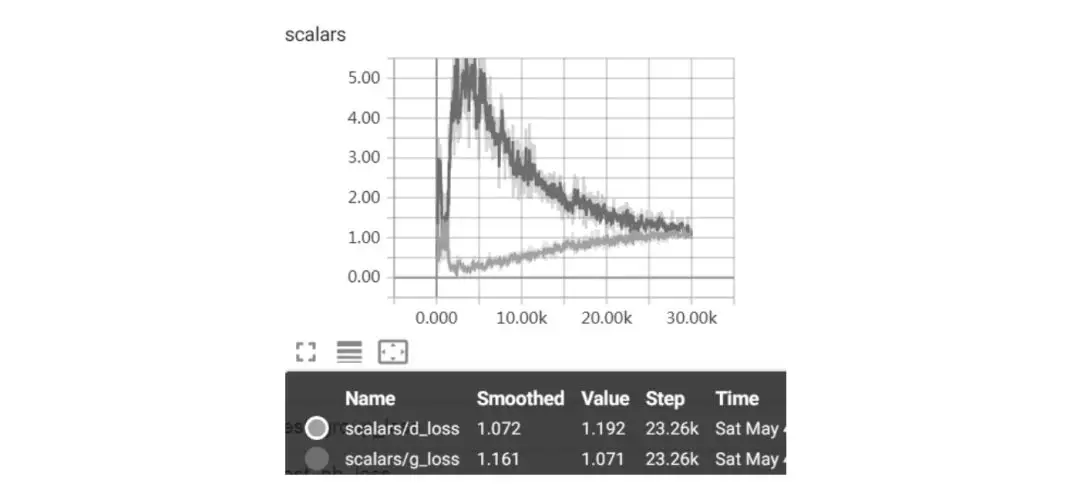## 8.6 DCGAN

DCGAN 在 GAN 的基础上优化了网络结构，加入了卷积层 ( Conv )、转置卷积 ( ConvTranspose )、批量正则 ( Batch_norm ) 等层，使得网络更容易训练，图 8-12 为使用卷积层的 DCGAN 的生成器网络结构示意图。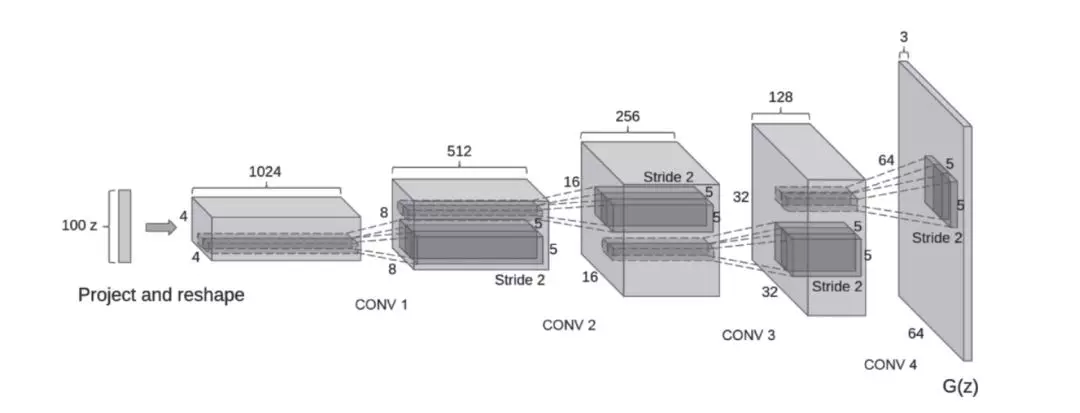pytorch-08-01.ipynb 代码中含有使用卷积层的实例，有兴趣的读者可参考一下。下面是使用卷积层的判别器及使用转置卷积的生成器的一个具体代码。

1. 使用卷积层、批规范层的判别器：

class Discriminator(nn.Module):    def __init__(self):        super(Discriminator, self).__init__()        self.main = nn.Sequential(            # 输入大致为 (nc) x 64 x 64，nc 表示通道数            nn.Conv2d(nc, ndf, 4, 2, 1, bias=False),            nn.LeakyReLU(0.2, inplace=True),            # ndf 表示判别器特征图的大小            nn.Conv2d(ndf, ndf * 2, 4, 2, 1, bias=False),            nn.BatchNorm2d(ndf * 2),            nn.LeakyReLU(0.2, inplace=True),nn.Conv2d(ndf * 2, ndf * 4, 4, 2, 1, bias=False),            nn.BatchNorm2d(ndf * 4),            nn.LeakyReLU(0.2, inplace=True),            nn.Conv2d(ndf * 4, ndf * 8, 4, 2, 1, bias=False),            nn.BatchNorm2d(ndf * 8),            nn.LeakyReLU(0.2, inplace=True), nn.Conv2d(ndf * 8, 1, 4, 1, 0, bias=False),            nn.Sigmoid()        )     def forward(self, input):        return self.main(input){1}
1. 使用转置卷积、批规范层的生成器：

class Generator(nn.Module):    def __init__(self):        super(Generator, self).__init__()        self.main = nn.Sequential(            # 输入 Z，nz 表示 Z 的大小。            nn.ConvTranspose2d( nz, ngf * 8, 4, 1, 0, bias=False),            nn.BatchNorm2d(ngf * 8),            nn.ReLU(True),            # ngf 为生成器特征图大小            nn.ConvTranspose2d(ngf * 8, ngf * 4, 4, 2, 1, bias=False),            nn.BatchNorm2d(ngf * 4),            nn.ReLU(True),            # state size. (ngf*4) x 8 x 8            nn.ConvTranspose2d( ngf * 4, ngf * 2, 4, 2, 1, bias=False),            nn.BatchNorm2d(ngf * 2),            nn.ReLU(True),            # state size. (ngf*2) x 16 x 16            nn.ConvTranspose2d( ngf * 2, ngf, 4, 2, 1, bias=False),            nn.BatchNorm2d(ngf),            nn.ReLU(True),            #nc 为通道数nn.ConvTranspose2d( ngf, nc, 4, 2, 1, bias=False),            nn.Tanh()            )     def forward(self, input):        return self.main(input){1}

## 8.7 提升 GAN 训练效果的一些技巧

1. 批量加载和批规范化，有利于提升训练过程中博弈的稳定性。

2. 使用 tanh 激活函数作为生成器最后一层，将图像数据规范在–1 和 1 之间，一般不用 sigmoid。

3. 选用 Leaky ReLU 作为生成器和判别器的激活函数，有利于改善梯度的稀疏性，稀疏的梯度会妨碍 GAN 的训练。

4. 使用卷积层时，考虑卷积核的大小能被步幅整除，否则，可能导致生成的图像中存在棋盘状伪影。